Courses

# NCERT Exemplar: Probability Notes | EduRev

## JEE : NCERT Exemplar: Probability Notes | EduRev

The document NCERT Exemplar: Probability Notes | EduRev is a part of the JEE Course JEE Revision Notes.
All you need of JEE at this link: JEE

SHORT ANSWER TYPE QUESTIONS

Q.1. If the letters of the word ALGORITHM are arranged at random in a row what is the probability the letters GOR must remain together as a unit?
Ans. Word ALGORITHM has 9 letters.
If GOR remain together, then it will remain together.
Number of letters = ALGORITHM = 6 + 1 = 7
Number of words = 7!
and the total number of words from ALGORITHM = 9!
So, the required probability =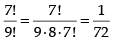Hence, the required probability = 1/72.

Q.2. Six new employees, two of whom are married to each other, are to be assigned six desks that are lined up in a row. If the assignment of employees to desks is made randomly, what is the probability that the married couple will have nonadjacent desks?
[Hint: First find the probability that the couple has adjacent desks, and then subtract it from 1.]
Ans. Number of desk occupied by one couple = 1
Only (4 + 1) = 5 persons to be assigned.
Number of ways of assigning these 5 persons = 5! x  2!
Total number of ways of assigning 6 persons = 6!
Probability that a couple has adjacent desk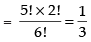So, the probability that the married couple will have no-adjacent desks =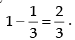Hence, the required probability = 2/3.

Q.3. Suppose an integer from 1 through 1000 is chosen at random,
find the probability that the integer is a multiple of 2 or a multiple of 9.
Ans. We have multiples of 2, from 1 to 1000 are
2, 4, 6, 8, ..., 1000
Let n be the number of terms
a = 2, d = 2, an = 1000
an = a + (n  1) d
1000 = 2 + (n – 1) 2
⇒ 1000 = 2n ⇒ n = 500
Now multiple of 9 from 1 to 1000 are
9, 18, 27, ..., 999
Here a = 9, d = 9 and am = 999 [m is the number of terms]
am = a + (m – 1) d
⇒ 999 = 9 + (m – 1) 9
⇒ 999 = 9m ⇒ m = 111
Now number multiples of 2 and 9 are
18, 36, 54, ..., 990
Here a = 18, ap = 990, d = 18  [p is the number of terms]
∴ ap = a + (p  1) d 990 = 18 + (p  1) 18
⇒ 990 = 18 + 18p – 18
18p =  990 ⇒ p = 55
⇒ Number of multiples of 2 or 9 = 500 + 111  55
= 556
Required probability =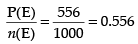Hence, the required probability = 0.556.

Q.4. An experiment consists of rolling a die until a 2 appears.
(i) How many elements of the sample space correspond to the event that the 2 appears on the kth roll of the die?
(ii) How many elements of the sample space correspond to the event that the 2 appears not later than the kth roll of the die?
[Hint:(a) First (k – 1) rolls have 5 outcomes each and kth rolls should result in 1 outcomes. (b)1 + 5 + 52 + ... + 5k–1.]
Ans. Number of sample space = 6
(i) Given that 2 appears on the kth roll of the die.
So first (k  1)th roll have 5 out comes each and kth roll results 2 i.e. only 1 outcome.
∴ Number of element of sample space correspond to the event that 2 appears on the kth roll of the die = 5k – 1.
(ii) In this case, 2  appears not later than kth roll of the die, then
it is possible that 2 comes in first throw i.e. 1 outcome. If 2 does not appear in first throw, then outcomes will be 5 and 2 outcomes in second throw i.e. 1 outcome.
∴ Possible out come = 5 x 1 = 5
Similarly, if 2 does not appear in second throw and appears in third throw
∴ Possible outcome = 5 x 5 x 1
Now we have the series:
= 1 + 5 + 5 x 5 + 5 x 5 x 5 +... + 5k - 1
= 1 + 5 + 52 + 53 + ...  + 5k - 1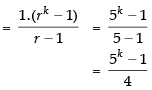Hence, the required answer =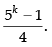Q.5. A die is loaded in such a way that each odd number is twice as likely to occur as each even number. Find P(G), where G is the event that a number greater than 3 occurs on a single roll of the die.
Ans. Given that probability of even numbers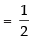x Probability of odd numbers
⇒ P(Odd) : P(Even) = 2 : 1
∴ P(odd number) =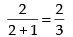and P(even number) =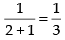Also given that, G the event that a number greater than 3 occurs in a single throw of die.
The possible outcome are 4, 5 and 6 out of which two are even and one is odd.
Required probability = P(G)
= 2 x P(even) x P(odd)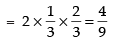Hence, the required probability is 4/9.

Q.6. In a large metropolitan area, the probabilities are .87, .36, .30 that a family (randomly chosen for a sample survey) owns a colour television set, a black and white television set, or both kinds of sets. What is the probability that a family owns either anyone or both kinds of sets?
Ans. Let E1 be the event that a family owns colour television set E2 be the event that the family owns black and white television set.
Given that P(E1) = 0.87, P(E2) = 0.36
and P(E1 E2) = 0.30
The probability that a family owns either colour television set or black and white television set
∴ P(E1 E2) =  P(E1) + P(E2) - P(E1 E2)
= 0.87 + 0.36 – 0.30
= 0.93
Hence, the required probability = 0.93.

Q.7. If A and B are mutually exclusive events, P (A) = 0.35 and P (B) = 0.45, find
(a) P (A′)
(b) P (B′)
(c) P (A ∪ B)
(d) P (A ∩ B)
(e) P (A ∩ B′)
(f) P (A′ ∩ B′)
Ans. Given that P(A) = 0.35 and P(B) = 0.45
Since the events A and B are mutually exclusive then P(A ∩ B) = 0
(a) P(A') = 1 - P(A) = 1 - 0.35 = 0.65
(b) P(B') = 1 - P(B) = 1 - 0.45 = 0.55
(c) P(A ∪ B) = P(A) + P(B) - P(A ∩ B) = 0.35 + 0.45 - 0
= 0.80
(d) P(A  B) = 0 [∴ A and B are mutually exclusive events]
(e) P(A ∩ B') =  P(A) - P(A ∩ B) = 0.35 - 0 = 0.35
(f) P(A' ∩ B') = 1 - P(A ∪ B) = 1 - 0.80 = 0.20.

Q.8. A team of medical students doing their internship have to assist during surgeries at a city hospital. The probabilities of surgeries rated as very complex, complex, routine, simple or very simple are respectively, 0.15, 0.20, 0.31, 0.26, .08. Find the probabilities that a particular surgery will be rated
(a) complex or very complex
(b) neither very complex nor very simple
(c) routine or complex
(d) routine or simple
Ans. Let E1, E2, E3, E4 and E5 be the events that the surgeries are rated as very complex, complex, routine, simple and very simple respectively.
P(E1) = 0.15, P(E2) = 0.20, P(E3) = 0.31, P(E4) = 0.26
and P(E5) = 0.08
(a) P(complex or very complex) = P(E1 or E2)
⇒ P(E1 E2) = P(E1) + P(E2) - P(E1 E2)
= 0.15 + 0.20  0
= 0.35 [∴ All event are independent]
(b) P(neither very complex nor Very simple) = P(E1' ∩ E5' )
= P(E1 E5)' = 1 - P(E1 E5)
= 1  [P(E1) + P(E5)]
= 1  [0.15 + 0.08] = 1  0.23 = 0.77
(c) P(routine or complex) = P(E3 or E2)
= P(E3 E2) = P(E3) + P(E2)
= 0.31 + 0.20 = 0.51
(d) P(routine or simple) = P(E3 E4)
= P(E3) + P(E4) = 0.31 + 0.26 = 0.57

Q.9. Four candidates A, B, C, D have applied for the assignment to coach a school cricket team. If A is twice as likely to be selected as B, and B and C are given about the same chance of being selected, while C is twice as likely to be selected as D, what are the probabilities that
(a) C will be selected?
(b) A will not be selected?
Ans. Given that A is twice as likely to be selected as B
i.e. P(A) = 2P (B)
and C is twice as likely to be selected as D
∴ P(C) = 2P(D) ⇒ P(B) = 2P(D)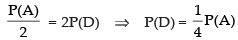Now B and C are given about the same chance
∴ P(B) = P(C)
Since, sum of all probabilities = 1
∴ P(A) + P(B) + P(C) + P(D) = 1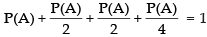⇒ 4P(A) + 2P(A) + 2P(A) + P(A) = 4
9P(A) = 4 ⇒ P(A) = 4/9
(a) P(C will be selected)  = P(C) = P(B) =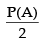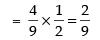(b) P(A will not be selected) = P(A') = 1 - P(A)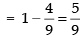Q.10. One of the four persons John, Rita, Aslam or Gurpreet will be promoted next month. Consequently the sample space consists of four elementary outcomes S = {John promoted, Rita promoted, Aslam promoted, Gurpreet promoted} You are told that the chances of John’s promotion is same as that of Gurpreet, Rita’s chances of promotion are twice as likely as Johns. Aslam’s chances are four times that of John.
(a) Determine P (John promoted)
P (Rita promoted)
P (Aslam promoted)
P (Gurpreet promoted)
(b) If A = {John promoted or Gurpreet promoted}, find P (A).
Ans. Let E1, E2, E3 and E4 be the events  that John promoted, Rita promoted, Aslam promoted and Gurpreet promoted respectively.
∴ Sample space S = {E1, E2, E3, E4}
Given that probability of John’s promotion is same as that of Gurpreet
∴ P(E1) = P(E4)
Rita’s chances of promotion are twice as likely as John
∴ P(E2) = 2P(E1)
and Aslam’s chances of promotion are 4 times that of John
∴ P(E3) = 4P(E1)
Since, the sum of all the probabilities = 1
∴ P(E1) + P(E2) + P(E3) + P(E4) = 1
⇒ P(E1) + 2P(E1) + 4P(E1) + P(E1) = 1
⇒ 8P(E1) = 1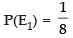(a) P (John promoted) = P(E1) = 1/8
P (Rita promoted) = P(E2) = 2P(E1) =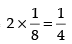P (Aslam promoted) = P(E3) = 4P(E1) =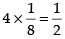P (Gurpreet promoted) = P(E4) = P(E1) = 1/8
(b) P(John promoted or Gurpreet promoted) = P(E1 E4)
⇒ P (E1 E4) = P(E1) + P(E4) - P(E1 E4)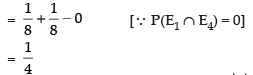Q.11. The accompanying Venn diagram shows three events, A, B, and C, and also the probabilities of the various intersections (for instance, P (A ∩ B) = .07). Determine

(a) P (A)
(b)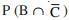(c) P (A ∪ B)
(d)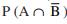(e) P (B ∩ C)
(f) Probability of exactly one of the three occurs.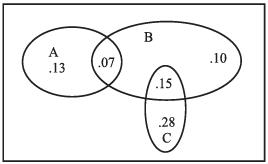Ans.
From the given Venn diagram
(a) P(A) = 0.13 + 0.07 = 0.20
(b)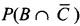= P(B) -  P(B  C)
= 0.07 + 0.10 + 0.15 - 0.15
= 0.07 + 0.10
= 0.17
(c) P(A ∪ B) = P(A) + P(B) - P(A ∩ B)
= 0.13 + 0.07 + 0.07 + 0.10 + 0.15 - 0.07
= 0.13 + 0.07 + 0.10 + 0.15
= 0.45
(d)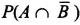= P(A) - P(A ∩ B) = 0.13 + 0.07 - 0.07 = 0.13
(e) P (B ∩ C) = 0.15
(f) P(exactly one of the three occurs) = 0.13 + 0.10 + 0.28
= 0.51

LONG ANSWER TYPE QUESTIONS

Q.12. One urn contains two black balls (labelled B1 and B2) and one white ball. A second urn contains one black ball and two white balls (labelled W1 and W2). Suppose the following experiment is performed. One of the two urns is chosen at random. Next a ball is randomly chosen from the urn. Then a second ball is chosen at random from the same urn without replacing the first ball.
(a) Write the sample space showing all possible outcomes
(b) What is the probability that two black balls are chosen?
(c) What is the probability that two balls of opposite colour are chosen?
Ans. Given that one of the two urns is chosen, then a ball is randomly chosen from the urn, then a second ball is chosen at random from the same urn without replacing the first ball
(a) Sample space S = {B1B2, B1W, B2B1, B2W, WB1, WB2, BW1, BW2, W1B, W1W2, W2B, W2W1}
Total number of Sample space, S = 12
(b) If two black balls are chosen then the favourable events are B1B2, B2B1 i.e. 2
∴ Required probability = 2/12 = 1/6
(c) If two balls of opposite colours are chosen then, the
required probability = 8/12 = 2/3

Q.13. A bag contains 8 red and 5 white balls. Three balls are drawn at random. Find the Probability that
(a) All the three balls are white
(b) All the three balls are red
(c) One ball is red and two balls are white
Ans. Given that: Number of red balls = 8
Number of white balls = 5
(a) P (all the three balls are white)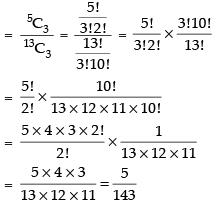(b) P (all the three balls are red)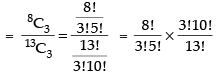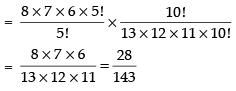(c) P (one ball is red and two balls are white)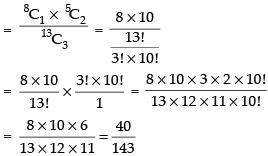Q.14. If the letters of the word ASSASSINATION are arranged at random. Find the Probability that
(a) Four S’s come consecutively in the word
(b) Two I’s and two N’s come together
(c) All A’s are not coming together
(d) No two A’s are coming together.
Ans. Total number of word is ASSASSINATION are 13.
Where, we have 3A’s, 4S’, 2I’s, 2N’s, 1T’s and 1O’s.
(a) If 4 S’s come consecutively in the word, then arrangement may be as follows: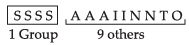Number of words when all S’s are together =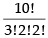and the total number of word formed from the words
ASSASSINATION =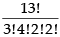∴ Required probability =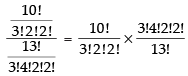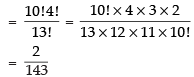(b) If 2I’s and 2N’s come together then there are 10 alphabets Number of words when 2I’s and 2N’s are come together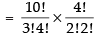∴ Required probability =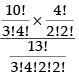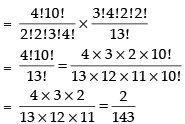(c) If all A’s are coming together, then three are 11 alphabets
Number of words when all A’s come together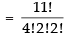Probability when all A’s come together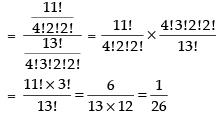Required probability when all A’s do not come together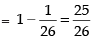(d) If no two A’s are together, then arranging the alphabets except A’s
– S  S  S  S  I – N  T  I  O – N
Number of ways of arranging all alphabets except A’s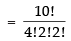There are 11 vacant places between these alphabets. ∴ 3 A’s can be placed in 11 places in 11C3 ways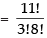Total number of words when no two A’s together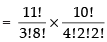Required probability =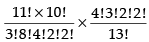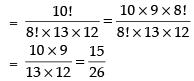Q.15. A card is drawn from a deck of 52 cards. Find the probability of getting a king or a heart or a red card.
Ans. Total number of cards = 52
Favourable events = 4 kings + 13 hearts + 26 red  13 – 2 = 28
Required probability = 28/52 = 7/13.

Q.16. A sample space consists of 9 elementary outcomes e1, e2, ..., e9 whose probabilities are
P(e1) = P(e2) = .08, P(e3) = P(e4) = P(e5) = .1
P(e6) = P(e7) = .2, P(e8) = P(e9) = .07
SupposeA = {e1, e5, e8}, B = {e2, e5, e8, e9
(a) Calculate P (A), P (B), and P (A ∩ B)
(b) Using the addition law of probability, calculate P (A ∪ B)
(c) List the composition of the event A ∪ B, and calculate P (A ∪ B) by adding the probabilities of the elementary outcomes.
(d) Calculate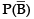from P(B), also calculatedirectly from the elementary outcomes of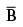Ans. Given that: S = {e1, e2, e3, e4, e5, e6, e7, e8, e9}
A = {e1, e5, e8} and B = {e2, e5, e8, e9}
P(e1) = P(e2) = 0.08
P(e3) = P(e4) = P(e5) = 0.1
P(e6) = P(e7) = 0.2, P(e8) = P(e9) = 0.07
(a) P(A) = P(e1) + P(e5) + P(e8)
= 0.08 + 0.1 + 0.07 = 0.25
(b) P(A  B) = P(A) + P(B) - P(A ∩ B) ...(i)
But P(B) =  P(e2) + P(e5) + P(e8) + P(e9)
= 0.08 + 0.1 + 0.07 + 0.07
= 0.32
and P(A ∩ B) = {e5, e8}
= P(e5) + P(e8) = 0.1 + 0.07 = 0.17
Putting the values in eq. (i) we get
P(A ∪ B) = 0.25 + 0.32 -  0.17
= 0.40
(c) A ∪ B = {e1, e2, e5, e8, e9}
P(A∪B) = P(e1) + P(e2) + P(e5) + P(e8) + P(e9)
= 0.08 + 0.08 + 0.1 + 0.07 + 0.07 = 0.40
(d)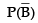= 1  P(B) = 1  0.32  = 0.68

Q.17. Determine the probability p, for each of the following events.
(a) An odd number appears in a single toss of a fair die.
(b) At least one head appears in two tosses of a fair coin.
(c) A king, 9 of hearts, or 3 of spades appears in drawing a single card from a well shuffled ordinary deck of 52 cards.
(d) The sum of 6 appears in a single toss of a pair of fair dice.
Ans.
(a) Possible outcomes of a single throw of die
S = {1, 2, 3, 4, 5, 6} out of which 1, 3, 5 are odd
Required probability = 3/6 = 1/2
(b) When a fair coin is tossed twice, then the sample space
S = {HH, HT, TH, TT}
Probability of getting atleast one head (HH, HT, TH)
= 3/4
(c) Favourable events are 4 kings + 2 of hearts + 3 of spades
= 4 + 1 + 1 = 6
= 6/52 = 3/26
(d) When a pair of dice is rolled, then total number of
sample space = 36 out of which (1, 5), (5, 1), (2, 4), (4, 2) and (3, 3) are the favourable events
Required probability =5/36.

OBJECTIVE ANSWER TYPE QUESTIONS

Q.18. In a non-leap year, the probability of having 53 tuesdays or 53 wednesdays is
(a) 1/7
(b) 2/7
(c) 3/7
(d) None of these
Ans. (a)
Solution.
There are 365 days in a non-leap year and there are 7 days in a week
365 ÷ 7  =  52 weeks + 1 day
So, this day may be Tuesday or Wednesday.
So, the required probability = 1/7.
Hence, the correct option is (a).

Q.19. Three numbers are chosen from 1 to 20. Find the probability that they are not consecutive
(a) 186 /190
(b) 187 /190
(c) 188 /190
(d)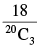Ans. (b)
Solution.
Set of three consecutive numbers from 1 to 20 are 1, 2, 3;
2, 3, 4; 3, 4, 5; ..., 18, 19, 20.
So, the probability that the numbers are consecutive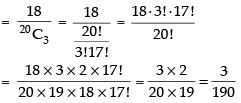P (three numbers are not consecutive)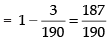Hence, the correct option is (b).

Q.20. While shuffling a pack of 52 playing cards, 2 are accidentally dropped. Find the probability that the missing cards to be of different colours.

(a) 29/52
(b) 1/2
(c) 26/51
(d) 27/51
Ans. (b)
Solution.
We know that out of 52 playing cards 26 are of red and 26 are of black colour.
P(both cards of different colour)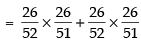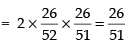Hence, the correct option is (c).

Q.21. Seven persons are to be seated in a row. The probability that two particular persons sit next to each other is
(a) 1/3
(b) 1/6
(c) 2/7
(d) 1/2
Ans. (c)
Solution.
The two particular persons to be seated next each other then, they form one group.
Now the permutation of 6 persons = 6! x 2!
and Total number of permutations of 7 persons = 7!
∴ Required probability =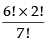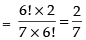Hence, the correct option is (c).

Q.22. Without repetition of the numbers, four digit numbers are formed with the numbers 0, 2, 3, 5. The probability of such a number divisible by 5 is
(a) 1/5
(b) 4/5
(c) 1/30
(d) 5/9
Ans. (d)
Solution.

Four digit number using the digits 0, 2, 3, 5 with out repetition and divisible by 5 with the given conditions is If unit place be filled with 0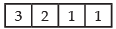Then the number of ways =    3 x 2 x 1 x 1 = 6
If unit place be filled with 5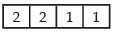Then the number of ways = 2 x 2 x 1 x 1 =
Total number of ways = 6 + 4 = 10
Total number of ways of arranging the digits 0, 2, 3, 5 to form
4-digit numbers without repetition is 3 x 3 x 2 x 1 = 18
Required probability = 10/18 = 5/9
Hence, the correct option is (d).

Q.23. If A and B are mutually exclusive events, then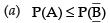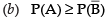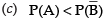(d) None of these
Ans. (a)
Solution.

For mutually exclusive events,
P(A ∩ B) = 0
∴ P(A ∪ B) = P(A) + P(B) [∵  P(A ∩ B) = 0]
⇒ P(A) + P(B) ≤ 1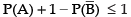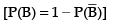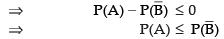Hence, the correct option is (a).

Q.24. If P (A ∪ B) = P (A ∩ B) for any two events A and B, then
(a) P (A) = P (B)
(b) P (A) > P (B)
(c) P (A) < P (B)
(d) None of these
Ans. (a)
Solution.

Given that:  P(A ∪ B) =  P(A ∩ B)
⇒ P(A) + P(B) - P(A ∩ B) = P(A ∩ B)
[P(A) – P(A ∩ B)] + [P(B) - P(A ∩ B)] = 0
But P(A)  P(A ∩ B)≥ 0 ...(i)
[∴ P(A ∩ B) ≤ P(A) or P(B)]
and P(B)  P(A ∩ B) ≥ 0 ...(ii)
From eq. (i) and (ii) we get P(A) = P(B)
Hence, the correct option is (a).

Q.25. If 6 boys and 6 girls sit in a row at random. The probability that all the girls sit together is
(a) 1/432
(b) 12/431
(c) 1/132
(d) None of these
Ans. (c)
Solution.
If all the girls sit together, then we consider it as 1 group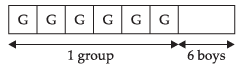Total number of arrangement of 6 + 1 = 7 persons in a row
= 7! and the girls also interchanged their places with 6! ways.
Required probability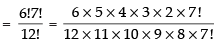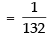Hence, the correct option is (c).

Q.26. A single letter is selected at random from the word ‘PROBABILITY’.
The probability that it is a vowel is
(a) 1/3
(b) 4/11
(c) 2/11
(d) 3/11
Ans. (b)
Solution.
Total number of alphabets in probability = 11
Number of vowels = 4
∴ Required probability = 4/11
Hence, the correct option is (b).

Q.27. If  the probabilities for A to fail in an examination is 0.2 and that for B is 0.3, then the probability that either A or B fails is
(a) > 0.5
(b) 0.5
(c) ≤ 0.5
(d) 0
Ans. (c)
Solution.
Given that: P(A fails) = 0.2
P (B fails) = 0.3
∴ P(either A or B fails) ≤ P(A fails) + P(B fails)
0.2 + 0.3
≤ 0.5
Hence, the correct option is (c).

Q.28. The probability that at least one of the events A and B occurs is 0.6. If A and B occur simultaneously with probability 0.2, then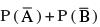is

(a) 0.4
(b) 0.8
(c) 1.2
(d) 1.6
Ans. (c)
Solution.

Given that: P(A ∪ B) = 0.6
P(A ∩ B) = 0.2
∴ P(A ∪ B) = P(A) + P(B) - P(A ∩ B)
⇒ 0.6 = P(A) + P(B) - 0.2
P(A) + P(B) = 0.6 + 0.2 = 0.8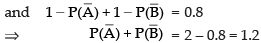Hence, the correct option is (c).

Q.29. If M and N are any two events, the probability that at least one of them occurs is
(a) P (M) + P (N) – 2P (M ∩ N)
(b) P (M) + P (N) – P (M ∩ N)
(c) P (M) + P (N) + P (M ∩ N)
(d) P (M) + P (N) + 2P (M ∩ N)
Ans. (b)
Solution.
If M and N are any two events, then
P(M ∪ N) = P(M) + P(N) - P(M ∩ N)
Hence, the correct option is (b).

TRUE OR FALSE STATEMENTS

Q.30. The probability that a person visiting a zoo will see the giraffee is 0.72, the probability that he will see the bears is 0.84 and the probability that he will see both is 0.52.
Ans. Given that:
P(to see giraffee) = 0.72
P(to see bears) = 0.84
P(to see both giraffee and bears) = 0.52
∴ P(to see giraffee or bear)
= P(to see giraffee) + P((to see bear) – P(to see both)
= 0.72 + 0.84 – 0.52
= 1.04 which is not possible.
Hence, the given statement is False.

Q.31. The probability that a student will pass his examination is 0.73, the probability of the student getting a compartment is 0.13, and the probability that the student will either pass or get compartment is 0.96.
Ans. Let E be the event that the student will pass and F be the event that he will get compartment
P(E) = 0.73, P(F) = 0.13 and P(E ∪ F) = 0.96
∴ P(E ∪ F) = P(E) + P(F) - P(E ∩ F)
= 0.73 + 0.13  0 [∴ P(E ∩ F) = 0]
= 0.86
But P(E ∩ F) = 0.96
Hence, the given statement is False.

Q.32. The probabilities that a typist will make 0, 1, 2, 3, 4, 5 or more  mistakes in typing a report are, respectively, 0.12, 0.25, 0.36, 0.14, 0.08, 0.11.
Ans. Sum of all probabilities = 1
P(0) + P(1) + P(2) + P(3) + P(4) + P(5)
= 0.12 + 0.25 + 0.36  + 0.14 + 0.08 + 0.11
= 1.06 > 1
Hence, the given statement is False.

Q.33. If A and B are two candidates seeking admission in an engineering College. The probability that A is selected is .5 and the probability that both A and B are selected is at most .3. Is it possible that the probability of B getting selected is 0.7?
Ans. Given that P(A) = 0.5 and P(A ∩ B) ≤ 0.3
Now P(A) x P(B) ≤ 0.3
0.5 x P(B) ≤ 0.3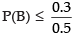⇒ P(B) ≤ 0.6
Hence, the given statement is False.

Q.34. The probability of intersection of two events A and B is always less than or equal to those favourable to the event A.
Ans. Here P(A ∩ B) ≤ P(A)
Which is always true.
Hence, given statement is True.

Q.35. The probability of an occurrence of event A is .7 and that of the occurrence of event B is .3 and the probability of occurrence of both is 0.4.
Ans. Here, P(A) = 0.7, P(B) =n0.3
P(A ∩ B) = P(A) x P(B)
= 0.7 x 0.3 = 0.21
But the given probability is 0.4.
Hence, the given statement is False.

Q.36. The sum of probabilities of two students getting distinction in their final examinations is 1.2.
Ans. Since, the two given events are not related to the same Sample space. ∴ The sum of probabilities of two students getting distinction in their final examinations may be 1.2.
Hence, the given statement is True.

FILL IN THE BLANKS

Q.37. The probability that the home team will win an upcoming football game is 0.77, the probability that it will tie the game is 0.08, and the probability that it will lose the game is _____.
Ans. P(loosing the game) =  1 – (0.77 + 0.08)
= 1 – 0.85 = 0.15
Hence, the value of the filler is 0.15.

Q.38. If e1, e2, e3, e4 are the four elementary outcomes in a sample space and P(e1) = .1, P(e2) = .5, P (e3) = .1, then the probability of e4 is ______.
Ans. We know that the sum of all probabilities = 1
∴ P(e1) + P(e2) + P(e3) + P(e4) = 1
0.1 + 0.5 + 0.1 + P(e4) = 1
⇒ 0.7 + P(e4) = 1
∴ P(e4) = 1  0.7 = 0.3
Hence, the value of the filler is 0.3.

Q.39. Let S = {1, 2, 3, 4, 5, 6} and E = {1, 3, 5}, then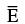is _________.
Ans. Given that: S = {1, 2, 3, 4, 5, 6}
E = {1, 3, 5}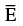= S  E = {2, 4, 6}
Hence, the value of the filler is {2, 4, 6}.

Q.40. If A and B are two events associated with a random experiment such that P ( A) = 0.3, P (B) = 0.2 and P (A ∩ B) = 0.1, then the value of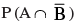is _______.
Ans. Given that: P(A) = 0.3, P(B) = 0.2
P(A ∩ B) = 0.1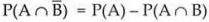= 0.3 – 0.1 = 0.2
Hence, the value of the filler is 0.2.

Q.41. The probability of happening of an event A is 0.5 and that of B is 0.3. If A and B are mutually exclusive events, then the probability of neither A nor B is ________.
Ans. Given that:  P(A) = 0.5, P(B) = 0.3
and P(A ∩ B) = 0
[∵ A and B are mutually exclusive events]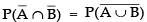= 1   P(A ∪ B)
= 1 – [P(A) + P(B)] = 1  (0.5 + 0.3)
= 1  0.8 = 0.2
Hence, the value of  the filler is 0.2.

Q.42. Match the proposed probability under Column C1 with the appropriate written description under column C2 :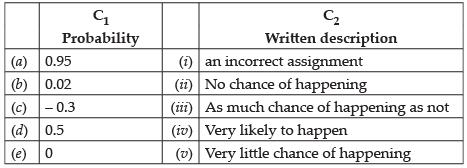Ans.
(i) 0.95 = Very likely to happen, so it is close to 1.
(ii) 0.02 = Very little chance of happening as the probability is very low.
(iii) – 0.3 = an incorrect assignment because probability is never negative.
(iv) 0.5 = as much chance of happening  as not because sum of chances of happening and not happening is one.
(v) 0 = no chance of happening.
Hence, (a) ↔ (iv), (b) ↔ (v), (c) ↔ (i), (d) ↔ (iii), (e) ↔ (ii)

Q.43. Match the following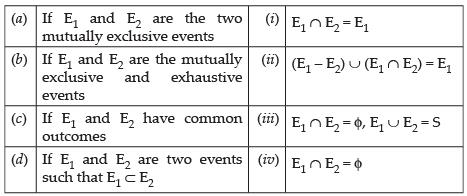Ans.
(a) If E1 and E2 are mutually exclusive events, then E1 E2 = ϕ.
(b) If  E1 and  E2 are mutually exclusive and exhaustive events then
E1 ∩ E2 = ϕ and E1 ∪ E2 = S.
(c) If E1 and E2 have common outcomes, then (E1 - E2) ∪ (E1 E2) = E1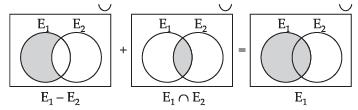(d) If E1 and Eare two events such that
E1 E2 ⇒ E1 E2 = E1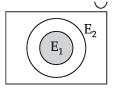Hence, (a)  (iv), (b)  (iii), (c)  (ii), (d)  (i)

Offer running on EduRev: Apply code STAYHOME200 to get INR 200 off on our premium plan EduRev Infinity!

233 docs

,

,

,

,

,

,

,

,

,

,

,

,

,

,

,

,

,

,

,

,

,

;